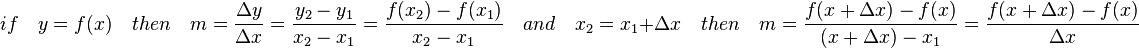# Difference quotient facts for kids

Kids Encyclopedia Facts

The difference quotient is a formula that finds the average rate of change of any function between two points. In calculus, the difference quotient is the formula used for finding the derivative which is the difference quotient between two points that are as close as possible which gives the rate of change of a function at a single point. The difference quotient was formulated by Isaac Newton.

## The Difference Quotient Defined

### A Simple Definition

The difference quotient can be described as the formula for finding the slope of a line that touches a curve at only two points (this line is called the secant line). If we are trying to find the slope of a perfectly straight line, then we use the slope formula which is simple the change in "y" divided by the change in "x". This is very accurate, but only for straight lines. The difference quotient, however, allows you to find the slope of any curve or line at any single point. The difference quotient, as well as the slope formula, is merely the change in "y" divided by the change in "x." The only difference is that in the slope formula, y is used as the y-axis, but in the difference quotient, the change in the y-axis is described by f(x). (For a detailed description, see the following section.)

### A Mathematical Definition

The difference quotient is the slope of the secant line between two points.

THE SLOPE FORMULA$if \quad y = f(x) \quad then \quad m= \frac{\Delta y}{\Delta x} = \frac{y_2-y_1}{x_2-x_1} = \frac{f(x_2) - f(x_1)}{x_2 - x_1} \quad and \quad x_2 = x_1 + \Delta x \quad then \quad m = \frac{f(x + \Delta x) - f(x)}{(x + \Delta x) - x_1} = \frac{f(x + \Delta x) - f(x)}{\Delta x}$

The difference quotient can be used to find the slope of a curve, as well as the slope of a straight line. After we find the difference quotient of a function, we have a new function, called the derivative. To find the slope of the curve or line we input the value of "x" and we get the slope. The process of finding the derivative via the difference quotient is called differentiation.

## Applications of the Difference Quotient (and the Derivative)

The derivative has many real life applications. One application of the derivative is listed below.

### Physics

In physics, the instantaneous velocity of an object (the velocity at an instance in time) is defined as the derivative of the position of the object as function of time. For example, if an object's position is given by x(t)=-16t2+16t+32, then the object's velocity is v(t)=-32t+16. To find instantaneous acceleration, take the derivative of the instantaneous velocity function. For example, in the above function, the acceleration function is a(t) = -32.Difference quotient Facts for Kids. Kiddle Encyclopedia.